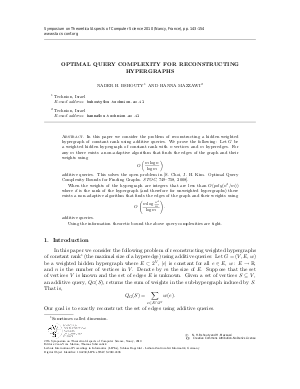Document# Optimal Query Complexity for Reconstructing Hypergraphs

### Authors Nader H. Bshouty, Hanna Mazzawi## File

LIPIcs.STACS.2010.2496.pdf
• Filesize: 305 kB
• 12 pages

## Cite As

Nader H. Bshouty and Hanna Mazzawi. Optimal Query Complexity for Reconstructing Hypergraphs. In 27th International Symposium on Theoretical Aspects of Computer Science. Leibniz International Proceedings in Informatics (LIPIcs), Volume 5, pp. 143-154, Schloss Dagstuhl - Leibniz-Zentrum für Informatik (2010)
https://doi.org/10.4230/LIPIcs.STACS.2010.2496

## Abstract

In this paper we consider the problem of reconstructing a hidden weighted hypergraph of constant rank using additive queries. We prove the following: Let $G$ be a weighted hidden hypergraph of constant rank with~$n$ vertices and $m$ hyperedges. For any $m$ there exists a non-adaptive algorithm that finds the edges of the graph and their weights using $$O\left(\frac{m\log n}{\log m}\right)$$ additive queries. This solves the open problem in [S. Choi, J. H. Kim. Optimal Query Complexity Bounds for Finding Graphs. {\em STOC}, 749--758, 2008]. When the weights of the hypergraph are integers that are less than $O(poly(n^d/m))$ where $d$ is the rank of the hypergraph (and therefore for unweighted hypergraphs) there exists a non-adaptive algorithm that finds the edges of the graph and their weights using $$O\left(\frac{m\log \frac{n^d}{m}}{\log m}\right).$$ additive queries. Using the information theoretic bound the above query complexities are tight.
##### Keywords
• Query complexity
• hypergraphs

## Metrics

• Access Statistics
• Total Accesses (updated on a weekly basis)
0